GeeksforGeeks App
Open AppBrowser
Continue

# Random Forest Approach in R Programming

Random Forest in R Programming is an ensemble of decision trees. It builds and combines multiple decision trees to get more accurate predictions. It’s a non-linear classification algorithm. Each decision tree model is used when employed on its own. An error estimate of cases is made that is not used when constructing the tree. This is called an out of bag error estimate mentioned as a percentage.

They are called random because they choose predictors randomly at a time of training. They are called forest because they take the output of multiple trees to make a decision. Random forest outperforms decision trees as a large number of uncorrelated trees(models) operating as a committee will always outperform the individual constituent models.

#### Theory

Random forest takes random samples from the observations, random initial variables(columns) and tries to build a model. Random forest algorithm is as follows:

• Draw a random bootstrap sample of size n (randomly choose n samples from training data).
• Grow a decision tree from bootstrap sample. At each node of tree, randomly select d features.
• Split the node using features(variables) that provide best split according to objective function. For instance, by maximizing the information gain.
• Repeat steps 1 to step 2, k times(k is the number of trees you want to create using subset of samples).
• Aggregate the prediction by each tree for a new data point to assign the class label by majority vote i.e pick the group selected by most number of trees and assign new data point to that group.

Example:
Consider a Fruit Box consisting of three fruits Apples, Oranges, and Cherries in training data i.e n = 3. We are predicting the fruit which is maximum in number in a fruit box. A random forest model using the training data with a number of trees, k = 3.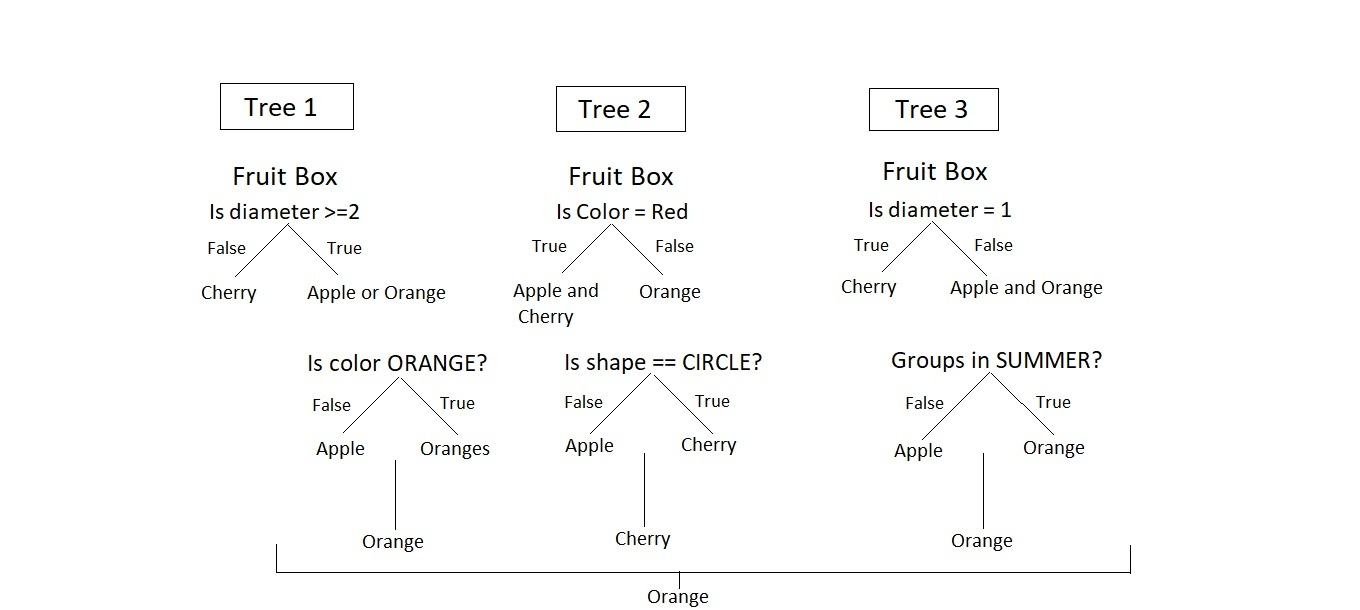The model is judged using various features of data i.e diameter, color, shape, and groups. Among orange, cheery, and orange, orange is selected to be maximum in fruit box by random forest.

#### The Dataset

`Iris` dataset consists of 50 samples from each of 3 species of Iris(Iris setosa, Iris virginica, Iris versicolor) and a multivariate dataset introduced by British statistician and biologist Ronald Fisher in his 1936 paper The use of multiple measurements in taxonomic problems. Four features were measured from each sample i.e length and width of the sepals and petals and based on the combination of these four features, Fisher developed a linear discriminant model to distinguish the species from each other.

 `# Loading data``data(iris)`` ` `# Structure ``str``(iris)`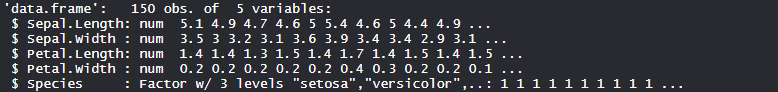#### Performing Random Forest on dataset

Using random forest algorithm on the dataset which includes 11 persons and 6 variables or attributes.

 `# Installing package``install.packages(``"caTools"``)       ``# For sampling the dataset``install.packages(``"randomForest"``)  ``# For implementing random forest algorithm`` ` `# Loading package``library(caTools)``library(randomForest)`` ` `# Splitting data in train and test data``split <``-` `sample.split(iris, SplitRatio ``=` `0.7``)``split`` ` `train <``-` `subset(iris, split ``=``=` `"TRUE"``)``test <``-` `subset(iris, split ``=``=` `"FALSE"``)`` ` `# Fitting Random Forest to the train dataset``set``.seed(``120``)  ``# Setting seed``classifier_RF ``=` `randomForest(x ``=` `train[``-``5``],``                             ``y ``=` `train\$Species,``                             ``ntree ``=` `500``)`` ` `classifier_RF`` ` `# Predicting the Test set results``y_pred ``=` `predict(classifier_RF, newdata ``=` `test[``-``5``])`` ` `# Confusion Matrix``confusion_mtx ``=` `table(test[, ``5``], y_pred)``confusion_mtx`` ` `# Plotting model``plot(classifier_RF)`` ` `# Importance plot``importance(classifier_RF)`` ` `# Variable importance plot``varImpPlot(classifier_RF)`

Output:

• Model classifier_RF: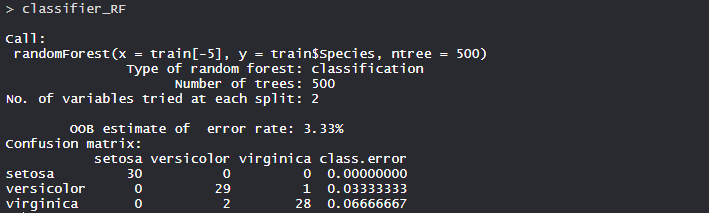The number of trees is 500 in the model and no. of variable tried at each split are 2. Classification error in setosa is 0.000 i.e 0%, Versicolor is 0.033 i.e 3.3% and virginica is 0.066 i.e 6.6% .

• Confusion matrix: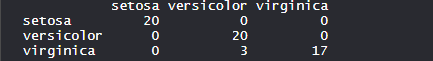So, 20 Setosa are correctly classified as Setosa. Out of 23 versicolor, 20 Versicolor are correctly classified as Versicolor, and 3 are classified as virginica. 17 virginica are correctly classified as virginica.

• Plot of model: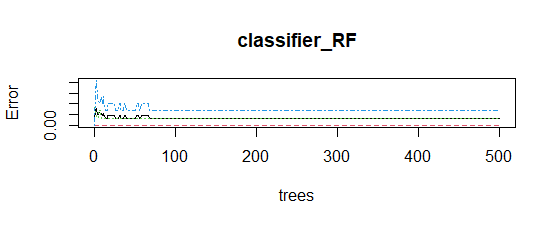Error rate is stabilized with an increase in the number of trees.

• Important features: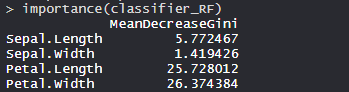Petal.Width is the most important feature followed by Petal.Length, Sepal.Width and Sepal.Length.

• Plot of important features: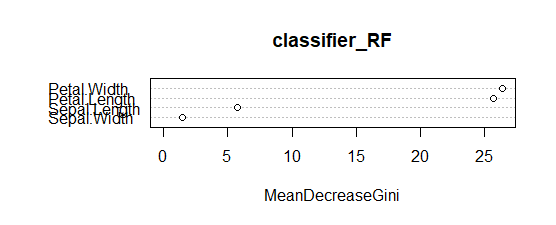The plot clearly shows Petal.Width as the most important feature or variable followed by Petal.Length, Sepal.Width and Sepal.Length.

So, random forest is a powerful algorithm used for classification in the industry.

My Personal Notes arrow_drop_up